Generalizing Two Structure Theorems of Lie Algebras to the Fuzzy Lie Algebras | OMICS International
Journal of Generalized Lie Theory and Applications

# Generalizing Two Structure Theorems of Lie Algebras to the Fuzzy Lie Algebras

Da Motta Ferreira JC* and Bruno Marietto MG

Center for Mathematics, Computation and Cognition, Federal University of ABC, 09210-170 Santo André, SP, Brazil

Corresponding Author:
Da Motta Ferreira JC
Center for Mathematics
Computation and Cognition
Federal University of ABC
09210-170 Santo André, SP, Brazil
Tel: +551149967914
E-mail: [email protected]

Received date: April 23, 2015; Accepted date: November 11, 2015; Published date: November 14, 2015

Citation: Da Motta Ferreira JC, Bruno Marietto MG (2015) Generalizing Two Structure Theorems of Lie Algebras to the Fuzzy Lie Algebras . J Generalized Lie Theory Appl 9:234. doi:10.4172/1736-4337.1000234

Copyright: © 2015 Da Motta Ferreira JC, et al. This is an open-access article distributed under the terms of the Creative Commons Attribution License, which permits unrestricted use, distribution, and reproduction in any medium, provided the original author and source are credited.

Visit for more related articles at Journal of Generalized Lie Theory and Applications

#### Abstract

In this paper we generalize two structure theorems of the class of Lie algebras to the class of fuzzy Lie algebras, namely the structure theorem of semisimple Lie algebras and the Levi’s decomposition theorem. Some open questions are also given.

#### Keywords

Semisimple fuzzy lie algebras; Levi’s fuzzy decomposition; Fuzzy lie algebras

#### Introduction

Lie algebras were proposed by Sophus Lie  and there are many applications of them in several branches of physics . The notion of fuzzy sets was introduced by Zadeh  and many mathematicians have been involved in extending the concepts and results of abstract Lie algebra to fuzzy theory. This paper is the continuation of the results obtained in , where we presented conditions to generalize the concepts of solvable and nilpotent radicals of Lie algebras (called of solvable and nilpotent fuzzy radicals, respectively) to a class of fuzzy Lie algebras. In this article we use the solvable fuzzy radical to generalize the structure theorem of semisimple Lie algebras and the Levi’s decomposition theorem to a class of the fuzzy Lie algebras. The results presented in this paper are still strongly connected with results proved in [5-10].

#### Fuzzy Sets, Fuzzy Lie Algebras and Fuzzy Lie Ideals

In this section we present the basic concepts of fuzzy sets, fuzzy Lie algebras, fuzzy ideals among others which will be used throughout this paper. More details referring to these concepts and its properties can be found in .

A mapping of a non-empty set X into the closed unit interval [0,1] is called a fuzzy set of X and the set {μ (x)| x ∈ X} is called the image of denoted by μ (X). For all real t∈ [0,1] the subset [μ]t = {x ∈ X| μ (x) ≥ t} is called a t-level set of μ and the set { x | x ∈ X, μ (x) > 0} is called the support of μ denoted by μ*.

A set S ⊂ [0,1] is said to be an upper well ordered set if for all non-empty subsets C ⊂ S, then supC∈C. One defines the set.

F(X, S) = {v | v is an fuzzy set of X such that v(X) ⊆ S}.

Let L be a Lie algebra over a field F. A fuzzy set μ of L is called a fuzzy Lie algebra of L if satisfies the following conditions: (i) μ (ax + by)μ (x) ∧ μ (y), (ii) μ (xy) ≥ μ (x) ∧ μ (y) and (iii)μ(0) = 1, for all a, b∈F and x, y∈L. A fuzzy set v of L is called a fuzzy subalgebra of μ if v is a fuzzy Lie algebra of L satisfying v(x) ≤ μ (x) for all x∈L. One has that μ is a fuzzy Lie algebra of L if, and only if, the t-level sets [μ]t are subalgebras of L, for all t∈]0,1]. Also, v is a fuzzy subalgebra of μ if, and only if, the t-level sets [v]t are subalgebras of [μ]t, for all t∈]0,1]. Moreover, if μ is a fuzzy algebra of L then μ* is a subalgebra of L.

A fuzzy set v of L is called a fuzzy ideal of L if satisfies the following conditions: (i) v (ax + by) ≥ v (x) ∧ v (y), (ii) v (xy) ≥ v (x) ∧ v (y) and (iii) )v(0) = 1, for all a, b∈F and x, y∈L. A fuzzy set v of L is called a fuzzy ideal of μ if v is a fuzzy Lie ideal of L satisfying v(x) ≤ μ (x) for all x∈L. One has that v is a fuzzy ideal of L if, and only if, the t-level sets [v]t are ideals of L, for all t∈]0,1]. Moreover, any fuzzy ideal of L is a fuzzy algebra of L, any fuzzy ideal of μ is a fuzzy subalgebra of μ and if v is a fuzzy ideal of L then v* is an ideal of L.

We define the null fuzzy algebra of μ as the fuzzy set of Land as a consequence of this definition we assume that our upper ordered set S has the real numbers 0 and 1.

A fuzzy Lie algebra μ of L is called abelian if μ2 = 0 and non-abelian otherwise.

If v1,…,vn are fuzzy sets of L, one defines: (i) The fuzzy set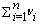L (sum) as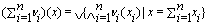for all x∈L. (ii) The fuzzy set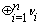of L (direct sum) as the fuzzy set sumsatisfying the condition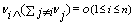, for all i=1,…,n, and (iii) The fuzzy set v1v2 of L (product) as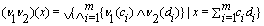for all x ∈ L.

To understand the main result of  which will be used at the end of this section and in the remainder of this article, we present the following definition.

For any fuzzy subalgebra v of a fuzzy algebra μ one defines inductively the derived series of v as the descending chain of fuzzy subalgebras of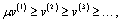by setting v(1) = v and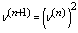for every n ≥ 1, and the lower central series of v as the descending chain of fuzzy algebras of μ v1 ≥v2 ≥v3…, by defying v1 = v and vn= vvn−1 for every n ≥ 2. The fuzzy subalgebra v is said solvable (resp., nilpotent) if there exists an integer k = k(v) ≥1 such that v(k) = 0 (resp., v(k) = 0).

Theorem 2.1. [4, Theorem 24] Let L be a finite dimensional Lie algebra over a field F and S an upper well ordered set. Then every solvable (resp., nilpotent) fuzzy ideal v of μ in F(L,S) is contained in a unique maximal solvable (resp., nilpotent) fuzzy ideal of μ in F(L,S), called solvable (resp., nilpotent) fuzzy radical of μ in F(L,S) and denoted by R (μ, S) (resp., N(μ, S)).

Let μ be a fuzzy Lie ideal of L. One says that μ is a simple fuzzy ideal if: (i) μ is a non-abelian fuzzy ideal and (ii) for all fuzzy ideals v of μ, one has either [v]t = [μ]t or [v]t = {0}, for all t∈]0,1].

To conclude this section, we extend the notion of a semisimple fuzzy ideal [4, Definition 26] for a semisimple fuzzy algebra.

Let L be a finite dimensional Lie algebra over a field F, S an upper well ordered set and μ a fuzzy algebra of L in F(L,S). One says that μ is a semisimple fuzzy algebra in F(L,S) if: (i) μ is a non-abelian fuzzy algebra and (ii) its solvable fuzzy radical in F(L,S) is o, that is, R (μ, S) = o.

#### Semisimple Fuzzy Ideals

In this section we generalize the theorem of decomposition of a semisimple Lie algebra as a direct sum of simple Lie ideals for the case of a semisimple fuzzy ideal, similarly to the crisp case. For this, we begin with the following definition.

Definition 3.1. Let L be a Lie algebra over a field F, S an upper well ordered set and μ a fuzzy ideal of L. One says that a fuzzy set π of L is a fuzzy ideal of μ relative to μ* if the following conditions are satisfied:

(i) πμ (and hence [π]t ⊆ [μ]t, for all t ∈]0,1]);

(ii) [π]t is an ideal of μ* for all t ∈]0,1].

In this case, π* is also an ideal of μ*.

If π is a fuzzy ideal of μ relative to μ*, then one says that a fuzzy set σ of L is a fuzzy ideal of π relative to μ* if the following conditions are satisfied:

(iii) σ ≤ π (and hence [σ]t ⊆ [π]t, for all t ∈]0,1]);

(iv) [σ]t is an ideal of π* for all t ∈]0,1].

In this case, σ* is also an ideal of π*.

One says that a fuzzy ideal π of μ relative to μ* is a simple fuzzy ideal of μ relative to μ* if the following conditions are satisfied:

(v) π is a non-abelian fuzzy ideal in μ* (that is, π2 ≠ o in μ*);

(vi) for all fuzzy ideal σ of π relative to μ*, one has either [σ]t = [π]t or [σ]t = {0} for all t ∈]0,1].

Hereafter, we exemplify each of the fuzzy ideals defined above.

Example 3.2. Let L be a finite dimensional Lie algebra over a field F,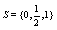M is an ideal of L and μ a fuzzy set of L defined by its t-level sets as: [μ]0 = L, [μ]t= M, for all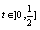and [μ]t= {0}, for all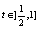. It is easy to check that S is an upper well ordered set and μ a fuzzy ideal of L in F(L,S) such that μ* = M. Next, from the representation of a fuzzy set, according to [4, Section 1], if P is an ideal of M, then the fuzzy set π of L defined by its t-level sets as:[π]0= L, [π]t= P, for all, and [π]t={0}, for all, is a fuzzy ideal of μ relative to μ* in F(L,S) such that π*= P. Yet, if S is an ideal of P, then the fuzzy set σ of L defined by its t-level sets as: [σ]0= L, [σ]t= S, for all, and [σ]t= {0}, for all, is a fuzzy ideal of π relative to μ* in F(L,S) such that σ* = S. Now, let us suppose that P is simple. Then P(2)(=PP) ≠ 0 which implies that there are nonzero elements a1,…,an,b1,…,bn in P such that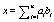is a nonzero element belongs to M(=μ*). It follows that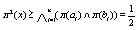, from the representation of a fuzzy set which shows that π is a non-abelian fuzzy ideal in μ*. Moreover, for all fuzzy ideal σ of π relative to μ*, we have [σ]t an ideal of π* (= P) for all t∈]0,1]. It follows that one has either [σ]t= P or [σ]t= {0} for all t∈]0,1] which implies one has either [σ]t= [π]t or [σ]t= {0} for all t∈]0,1]. Thus, π is is a simple fuzzy ideal of μ relative to μ*.

Theorem 3.3. Let L be a finite dimensional Lie algebra over a field F, μ a fuzzy Lie ideal of L and π a non-abelian (in μ*) fuzzy ideal of μ relative to μ*. If π is a simple fuzzy ideal of μ relative to μ*, then π* is not a solvable ideal of μ*.

Moreover, π is a simple fuzzy ideal of μ relative to μ* if, and only if, π* is a simple ideal of μ*.

Proof. First, let us observe that (π* )(2) is not a zero ideal of μ* since π2 is non-null in μ*. Since L is finite dimensional, there is a finite set of real numbers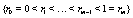such that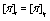for all t ∈ ] ri-1 , ri] (i = 1, ..., m), by . This implies that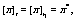for all t ∈]0,r1]. If π* is a solvable ideal of μ*, then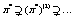and there is an integer k ≥ 1 such that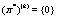Thus, by Negoita-Ralescu representation [4, Theorem 2.10], we can construct the fuzzy set σ of L defined by t-level sets: [σ]0= L, [σ]t = ( π* )(2) t σ π for all t ∈]0,r1], and [σ]t = {0} for all t ∈]r1,1]. It follows that σ is a solvable fuzzy ideal of π relative to μ* such that [σ]t ≠ [π]t or {0} for some t∈]0, r1]. This is an absurd.

Now let us consider J an ideal of π*. Again, by Negoita-Ralescu representation [7, Theorem 2.10], let us consider the fuzzy set σ of L defined by t-level sets: [σ]0 = L, [σ]t = J, for all t ∈]0,r1], and [σ]t = {0} for all t ∈]r1,1]. Clearly, σ is a fuzzy ideal of π relative to μ* and so we have either [σ]t = [π]t or [σ]t= {0}, for all t ∈]0,1]. It follows that J=π* or J={0}. So π* is a simple ideal of μ*. Reciprocally, let us consider σ a fuzzy ideal of π relative to μ*. Then [σ]t ⊆ [π]t and the t-level set [σ]t is an ideal of π*, for all t ∈[0,1]. Since π* is a simple ideal, then either [σ]t = π* or [σ]t = {0}, for all t ∈]0,1]. This implies that [σ]t = [π]t or [σ]t = {0}, for all t ∈]0,1]. So π is a simple fuzzy ideal of relative to μ*.

Theorem 3.4. Let L be a finite dimensional Lie algebra over a field F of the characteristic 0, S an upper well ordered set and μ a non-abelian fuzzy ideal of L in F(L,S). Then μ is semisimple in F(L,S) if, and only if, μ* is a semisimple ideal of L.

Proof. Firstly, let us observe that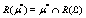, where R(μ*) and R(L) are the radicals of μ* and L, respectively, by [12, Theorem 3.7]. Since R(μ*) is a solvable ideal of L contained in μ*, then R(μ*) = {0}, by [4, Theorem 5.6]. Thus μ* is a semisimple ideal of L. Reciprocally, is immediate that R(μ, S) = o, by [12,Theorem 3.7] again.

Theorem 3.5. Let L be a finite dimensional Lie algebra over a field F of the characteristic 0, S an upper well ordered set and μ a non-abelian fuzzy ideal of L in F(L,S). If μ is semisimple in F(L,S), then there are simple fuzzy ideals v1,…,vn of μ relative to μ* in F(L,S) such that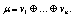Moreover:

(i) for every simple fuzzy ideal π of μ relative to * there is a unique fuzzy ideal vi (1 ≤ i ≤ n) such that π* = ν*i

(ii) for each fuzzy ideal π of μ relative to μ* there are fuzzy ideals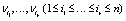such that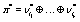.

Proof. From the hypothesis of the Theorem, we have that μ* is an ideal of L which is a semisimple algebra, by Theorem 3.4. Hence,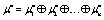for simple ideals of μ*, where every simple ideal of μ* coincides with one of the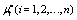and each ideal of μ* is a sum of certain simple ideals of μ*, by [1,Theorem and Corollary,5.2,pp. 23]. This implies that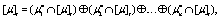where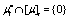or μ*i , for all i ∈]0,1], since [μ]t is an ideal of μ*. By Negoita-Ralescu representation [7, Theorem 2.10], there are unique fuzzy sets v1,….,vn of L such that the t-level sets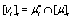(i =1,…,n) for all t∈]0,1], which implies that the fuzzy sets vi are fuzzy ideals of μ relative to μ*. As L is finite dimensional, there is a finite set of real numbers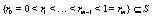such that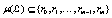and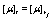for all t ∈]rj−1,rj](j = 1,…,m). It follows that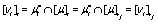(i =1,…,n) for all t ∈]rj−1, rj](i=1,…,m) which results in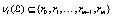, from the representation of the fuzzy sets vi in terms of its t-level sets [vi]t as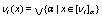(i =1,…,n), by [13,14]. This shows that v1,….,vn are fuzzy ideals in F(L,S). Yet, for all fuzzy ideal σi of vi relative to *(i =1,…,n), we have [σi]t ⊂ [vi]t and [σi]t is an ideal of vi* (= μ*i) for all t ∈]0,1]. Hence, one has either [σi]t = [vi]t or [σi]t = 0 for all t ∈]0,1], which shows that vi is simple.

Next, let us show that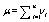. For an arbitrary x ∈ L, let us take (i) = α. If α = 0, then x ∉ μ*.Hence for all sum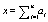, there is at least one index i such that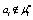which implies vi (ai) = 0. It follows that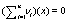Otherwise, if α ≠ 0, then writing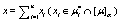we have x ∈ [μ]α and xi ∈ [vi]α (i =1,…,n), which yields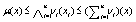. Also, since each vi (i =1,…,n) is a fuzzy ideal of μ relative to μ* we have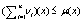. This shows that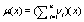allowing us to conclude. To show that this sum is direct, let us show that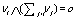for all i =1,…,n. Firstly, let us observe that it is sufficient to consider only the case when x ∈ μ*. Hence, let us fix an index i(1 ≤ I ≤ n) and let us consider an element x (≠ 0) ∈ μ*. If x∈μi*, then for all sum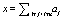there is at least one index j such that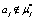which implies vj(aj) = 0. It follows that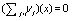which yields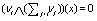. Otherwise, if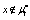then vi (x) = 0 which results in. Since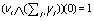then we conclude thatfor all i = 1,…,n. Consequently,Now, let π be a simple fuzzy ideal of μ relative to μ*. Then π* is a sum of certain simple ideals of μ*, by [1, Theorem and Corollary, 5.2, pp. 23]. It follows that there is a unique ideal μ*i (1 ≤ in ) such that π* = μ*i , by Theorem [1, Theorem and Corollary, 5.2, pp. 23] again. Finally, let π be a fuzzy ideal of μ relative to μ*. Then we have π* an ideal of μ* and therefore a sum of certain simple ideals of μ*, by [1, Theorem and Corollary, 5.2, pp. 23]. Hence there are fuzzy idealssuch that.

#### Levi Fuzzy Decomposition

In this section we generalize the theorem of Levi decomposition of a Lie algebra for the case of a class of fuzzy ideal, similarly to the crisp case.

Definition 4.1. Let L be a finite dimensional Lie algebra over a field F, S an upper well ordered set and μ a fuzzy ideal of L in F(L,S). One says that μ has Levi’s hereditary if there is a semisimple subalgebra v* of L such that:

(i)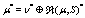;

(ii)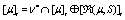for all t ∈]0,1].

In the following example we show that the conditions of the Definition 4.1 are not artificial.

Example 4.2. Let L be a finite dimensional Lie algebra over a field F of the characteristic 0,and μ a fuzzy set of L defined by its t-level sets as: [μ]t = L for all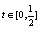and [μ]t = {0} for all. It is easy to check that S is an upper well ordered set and μ a fuzzy ideal of L in F(L, S) such that μ*= L. Also, [R(μ, S)]0 = L, [R(μ, S)]t = R(L) for alland [R(μ, S)]t = {0} for all, resulting in R(μ, S)*= R(L), where R(L) is the radical of L. Moreover, there is a semisimple subalgebra v* of L such that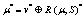by [10, Levi' s theorem], which implies v*∩ [μ]t = v* for all, v*∩ [μ]t = {0} for all. Thus, has Levi’s hereditary.

Theorem 4.3. Let L be a finite dimensional Lie algebra over a field F, S an upper well ordered set and μ a fuzzy ideal of L in F(L,S). If μ has Levi’s hereditary, then there exists a semisimple fuzzy subalgebra v of μ in F(L,S) such that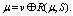Proof. From the hypothesis of the Theorem, there is a unique fuzzy set v of L such that [vIt = v*∩ [μ]t for all t ∈]0,1], by Negoita- Ralescu representation , Theorem 2.10]. The t-level sets [v]t are fuzzy subalgebras of L which implies that the fuzzy set v is a fuzzy subalgebra of μ satisfying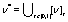. Now, using an argument quite similar to the one used in the Theorem 3.5, we can also conclude that v is in F(L, S). Furthermore, since v* is a semisimple subalgebra of L, then [R(v,S)]t = {0} for all t ∈]0,1]. This implies that R(v,S) = o and so v is semisimple. Let us show that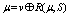. For an arbitrary x∈L, let us take μ(x) = α. If α = 0 , then x∉μ*. Hence for all sum x = a1 + a2, we have either a1 ∉ v* or a2 ∉ R(μ, S)*, by (i). It follows that v (a1) = 0 or R(μ, S)(a2) = 0 which results in v (a1) ∧ R(μ, S)(a2) = 0. So. Otherwise, if α ≠ 0, then x ∈ [μ]α which yields that there are elements a1 ∈ v* ∩ [μ]α = [v]α and a2 ∉ [R(μ, S)]α such that x = a1 + a2. Hence, μ (x) ≤ v (a1) ∧ R(μ, S)(a2) ≤ (v + R(μ, S))(x). Also, since v is a fuzzy subalgebra of μ and R(μ, S) is a fuzzy ideal of μ, then v(a1) ≤ μ(a1) and (R(μ, S))(a2) ≤ μ (a2) for arbitrary elements of L. It follows that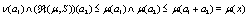for all sum x = a1 + a2, which implies (v + R(μ, S))(x) ≤ μ (x). This shows that μ = v + R(μ, S). To show that this sum is direct, let us show that v ∧ R(μ, S) = o. Firstly, let us observe that it is sufficient to consider only the case when x∈μ*. Hence, let us consider an element x (≠ 0) ∈μ*. If x ∈ v*, then x ∉ R(μ, S)* which implies R(μ, S)(x) = 0. It follows that (v∧ R(μ, S))(x) = 0. Since (v ∧ R(μ, S))(0) = 1, then we conclude that v ∧ R(μ, S) = o. Consequently,.

Example 4.4. From the Theorem 4.3 we can conclude that in the Example 4.2 there exists a semisimple fuzzy subalgebra v of μ in F(L, S) such that.

#### Open Questions

The history of the class of fuzzy Lie algebras proposed in  and in this paper is far from over. In fact, there are many unanswered questions and we list some of them as follows:

Question 5.1 Find a fuzzy version of Malcev-Harish-Chandra’s Theorem.

Question 5.2 Is it possible a fuzzy representation theory for the structure theory proposed?

Question 5.3 Perspectives for applications in Physics. Reviewing the history of applications of Lie algebras in Physics since its origin, is it possible to determine methods based on the fuzzy Lie algebras presented in this work, to be applied in the Particle Physics?

#### Acknowledgement

The authors would like to thank the referee for the valuable comments and suggestions.

#### References

Select your language of interest to view the total content in your interested language

### Article Usage

• Total views: 8705
• [From(publication date):
December-2015 - Dec 12, 2019]
• Breakdown by view type
• HTML page views : 8466Can't read the image? click here to refresh